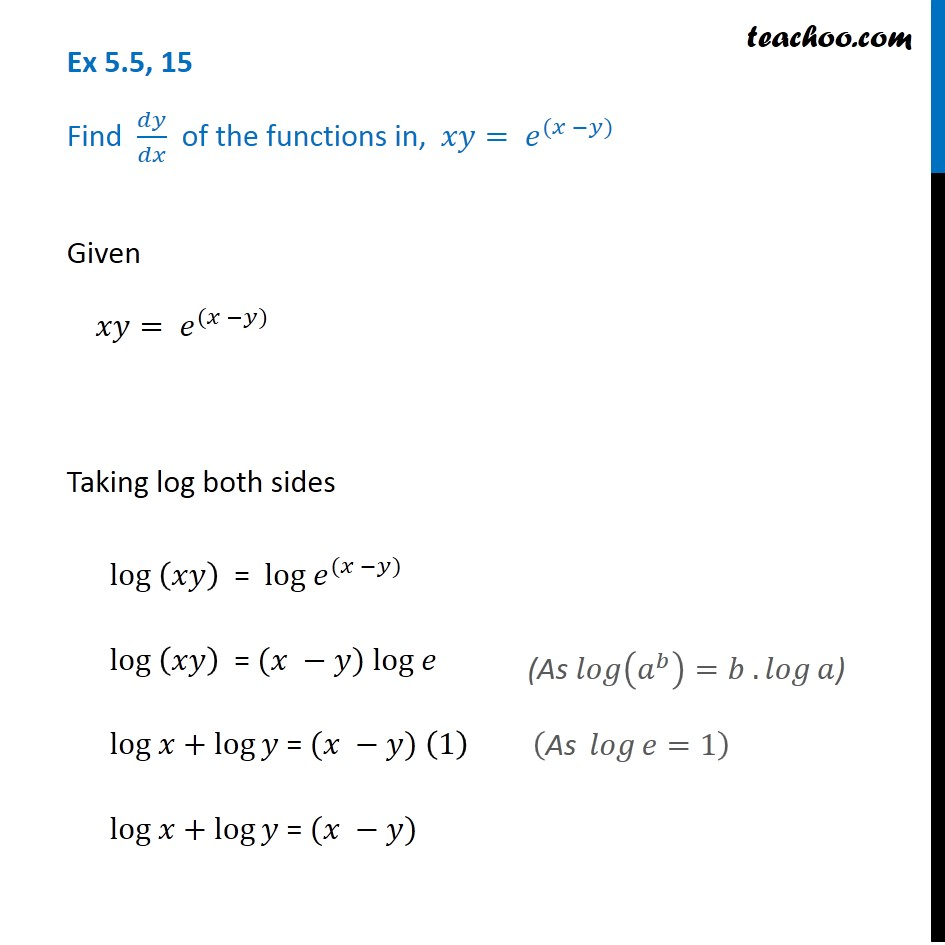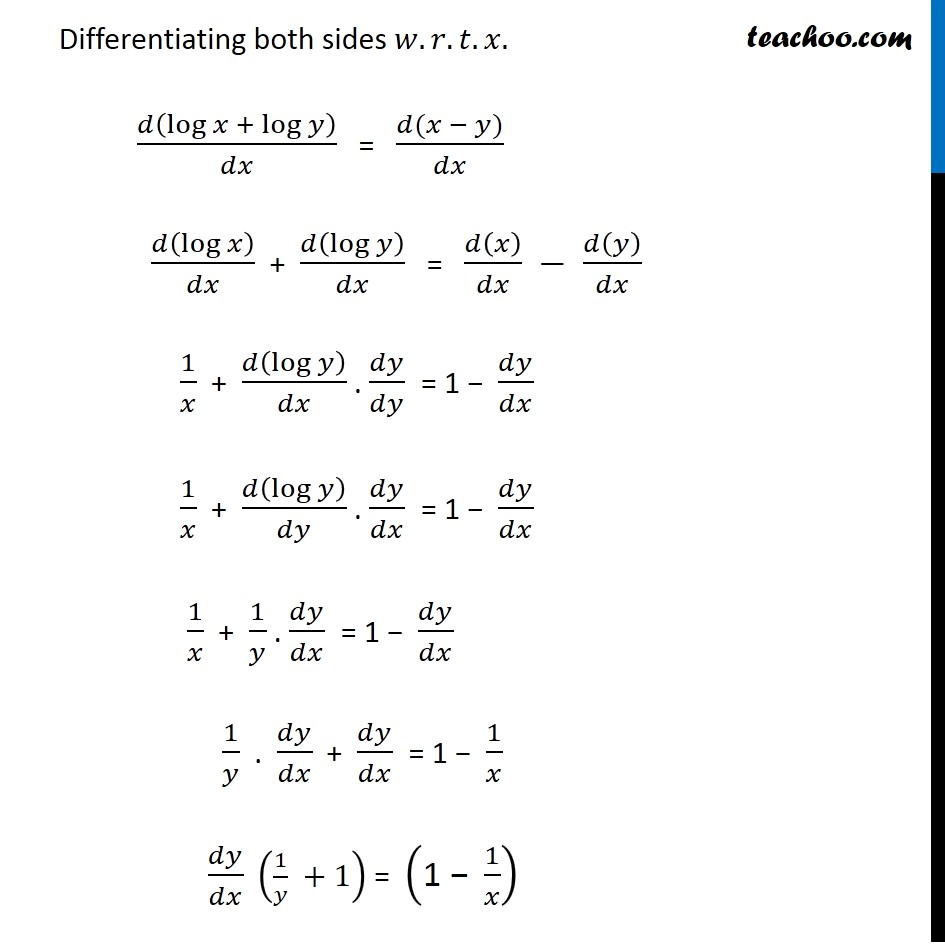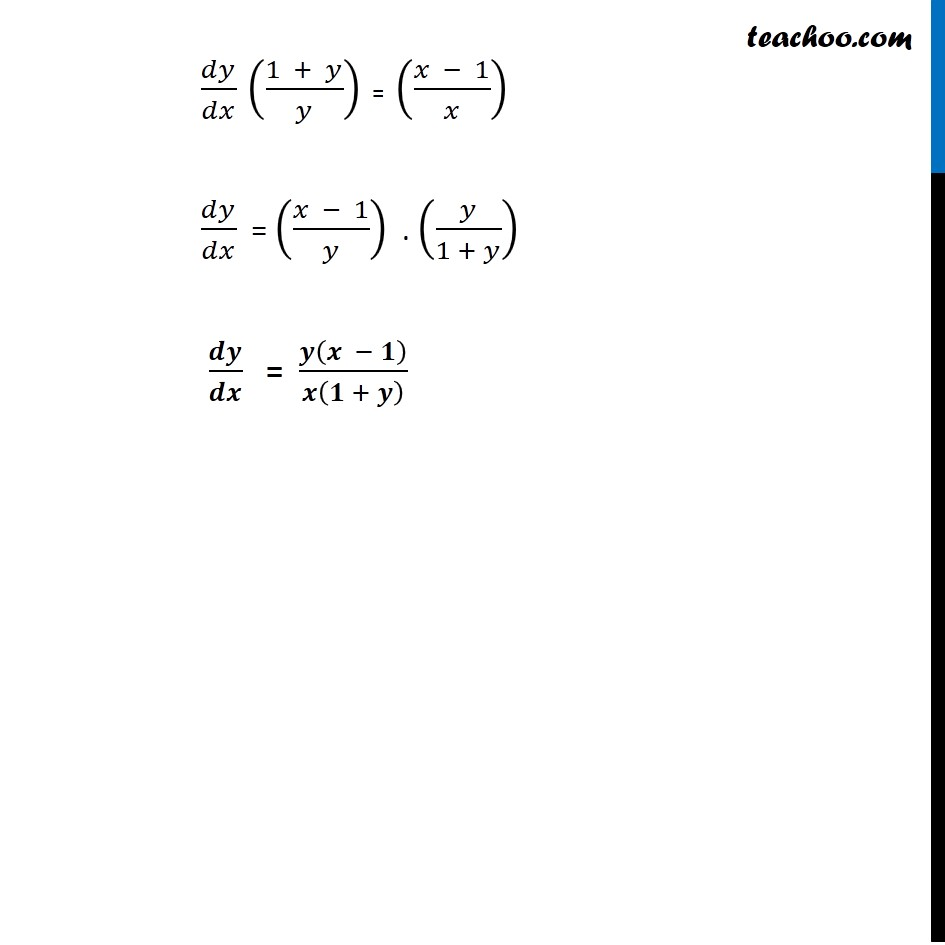Ex 5.5

Chapter 5 Class 12 Continuity and Differentiability
Serial order wiseLearn in your speed, with individual attention - Teachoo Maths 1-on-1 Class

### Transcript

Ex 5.5, 15 Find 𝑑𝑦/𝑑𝑥 of the functions in, 𝑥𝑦= 𝑒^((𝑥 −𝑦))Given 𝑥𝑦= 𝑒^((𝑥 −𝑦)) Taking log both sides log (𝑥𝑦) = log 𝑒^((𝑥 −𝑦)) log (𝑥𝑦) = (𝑥 −𝑦) log 𝑒 log 𝑥+log⁡𝑦 = (𝑥 −𝑦) (1) log 𝑥+log⁡𝑦 = (𝑥 −𝑦) (As 𝑙𝑜𝑔⁡(𝑎^𝑏 )=𝑏 . 𝑙𝑜𝑔⁡𝑎) Differentiating both sides 𝑤.𝑟.𝑡.𝑥. 𝑑(log 𝑥 + log⁡𝑦 )/𝑑𝑥 = (𝑑(𝑥 − 𝑦))/𝑑𝑥 𝑑(log 𝑥)/𝑑𝑥 + 𝑑(log⁡𝑦 )/𝑑𝑥 = 𝑑(𝑥)/𝑑𝑥 − 𝑑(𝑦)/𝑑𝑥 1/𝑥 + 𝑑(log⁡𝑦 )/𝑑𝑥 . 𝑑𝑦/𝑑𝑦 = 1 − 𝑑𝑦/𝑑𝑥 1/𝑥 + 𝑑(log⁡𝑦 )/𝑑𝑦 . 𝑑𝑦/𝑑𝑥 = 1 − 𝑑𝑦/𝑑𝑥 1/𝑥 + 1/𝑦 . 𝑑𝑦/𝑑𝑥 = 1 − 𝑑𝑦/𝑑𝑥 1/𝑦 . 𝑑𝑦/𝑑𝑥 + 𝑑𝑦/𝑑𝑥 = 1 − 1/𝑥 𝑑𝑦/𝑑𝑥 (1/𝑦 +1) = ("1 − " 1/𝑥) 𝑑𝑦/𝑑𝑥 ((1 + 𝑦)/𝑦) = ((𝑥 − 1)/𝑥) 𝑑𝑦/𝑑𝑥 = ((𝑥 − 1)/𝑦) . (𝑦/(1 + 𝑦)) 𝒅𝒚/𝒅𝒙 = 𝒚(𝒙 − 𝟏)/𝒙(𝟏 + 𝒚)